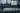# Four Sum - Leet Code Solution

November 17, 2020## Problem Statement

Given an array nums of n integers and an integer target, are there elements a, b, c, and d in nums such that a + b + c + d = target? Find all unique quadruplets in the array which gives the sum of target.

Notice that the solution set must not contain duplicate quadruplets.

``````Input: nums = [1,0,-1,0,-2,2], target = 0
Output: [[-2,-1,1,2],[-2,0,0,2],[-1,0,0,1]]

Input: nums = [], target = 0
Output: []``````

## Solution

Lets take help of our two-sum problem.

## Code

``````private List<List<Integer>> twoSum(int[] nums, int target, int start) {
List<List<Integer>> res = new ArrayList<>();
int l = start;
int r = nums.length-1;
while (l < r) {

/**
* second conditions are for avoiding duplicates.
*/

if (nums[l] + nums[r] < target || (l > start && nums[l-1] == nums[l])) {
l++;
}
else if (nums[l] + nums[r] > target || (r < nums.length-1 && nums[r] == nums[r+1])) {
r--;
}
else {
List<Integer> t = new ArrayList<>();
l++;
r--;
}
}

return res;
}

// -2 -1 0 0 1 2
private List<List<Integer>> helper(int[] nums, int target, int startIndex, int k) {
List<List<Integer>> res = new ArrayList<>();

if (startIndex >= nums.length || nums[startIndex] * k > target || target > nums[nums.length - 1] * k) {
return res;
}
else if (k == 2) {
return twoSum(nums, target, startIndex);
}
else {
for (int i=startIndex; i<nums.length; i++) {
//This condition is to avoid duplicates
if (i == startIndex || nums[i] != nums[i-1]) {
List<List<Integer>> res2 = helper(nums, target-nums[i], i+1, k-1);
for (List<Integer> t : res2) {
List<Integer> newRes = new ArrayList<>();

}
}
}
}

return res;
}

public List<List<Integer>> fourSum(int[] nums, int target) {
//sorting will help in avoiding duplicates in easy manner
Arrays.sort(nums);

return helper(nums, target, 0, 4);
}
``````

O(n^2)